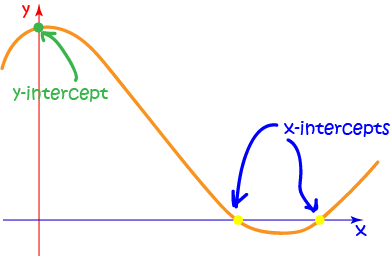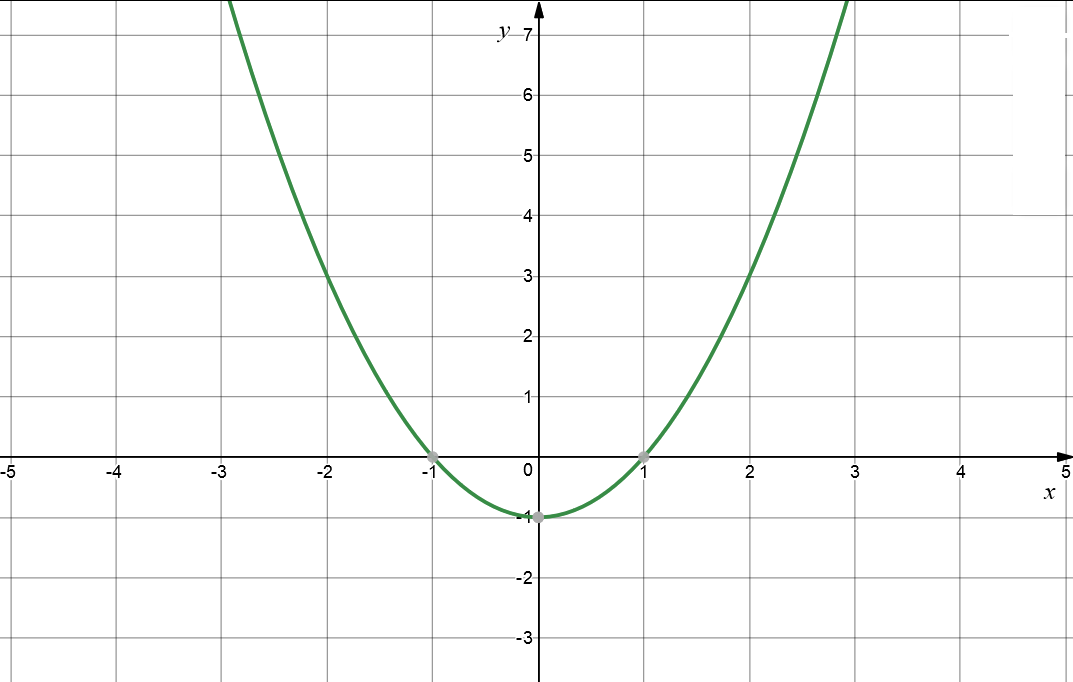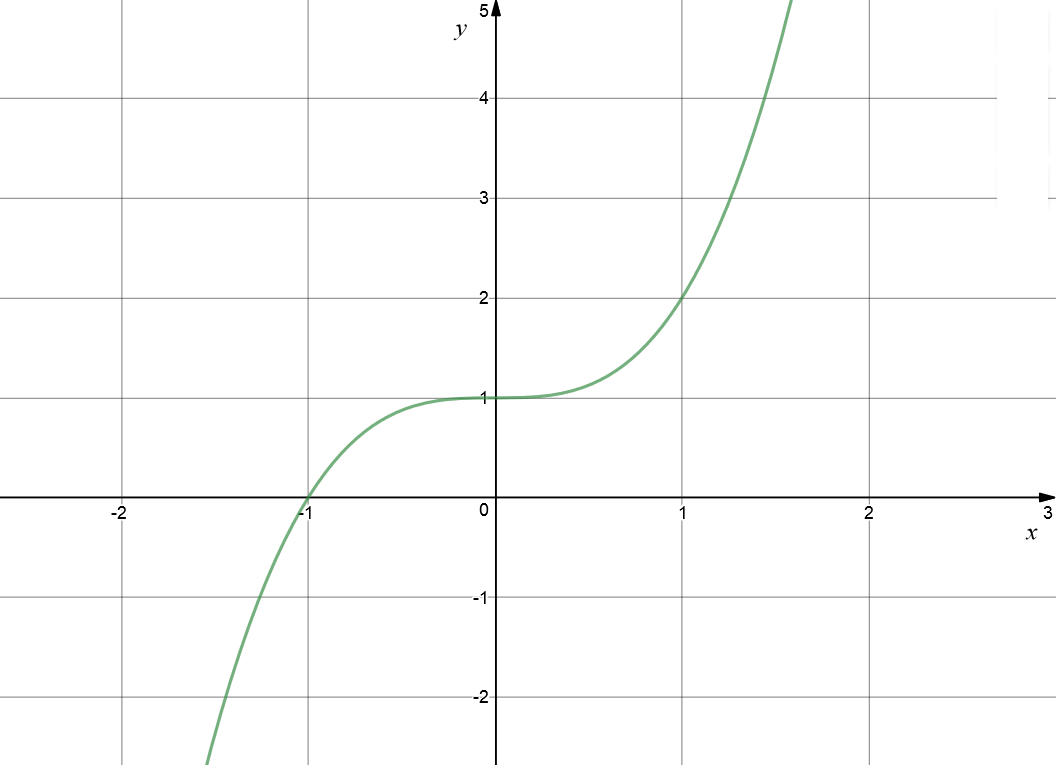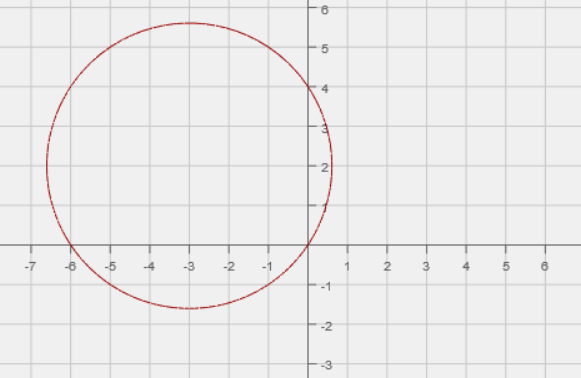Pull Down & Release to Refresh

# Using the Equation to Find the Intercepts

## What are Intercepts?

Intercepts are places where the graph of a function cuts the axes. As there are two axes in the $xy$-plane, there are two different types of intercepts:

• $x$-intercepts occur when the graph crosses the $x$-axis.
• $y$-intercepts occur when the graph crosses the $y$-axis.## Finding the Intercepts

The $x$-intercepts lie on the $x$-axis, so their coordinates have the form $(x,0)$.

To find the $x$-intercepts, set $y=0$ and solve for $x$.

The $y$-intercepts lie on the $y$-axis, so their coordinates have the form $(0,y)$.

To find the $y$-intercepts, set $x=0$ and solve for $y$.

## Examples

### Example 1

Find the intercepts of the function $y= x^2 - 1$.

Solution:

The $x$-intercepts occur when y = 0:

\begin{align*} x^2 - 1 &= 0\\ x^2 &= 1\\ x &= 1 \text{ or } -1 \end{align*}
The $x$-intercepts are at $(1,0)$ and $(-1.0)$.

The $y$-intercepts occur when x = 0:

\begin{align*} y &= x^2 - 1\\ y &= 0^2 -1\\ y &= -1 \end{align*}
The $y$-intercept is at $(0,-1)$ .

The graph looks like:### Example 2

Find the intercepts of the function $y= x^3 + 1$.

Solution:

The $x$-intercepts occur when y = 0:

\begin{align*} x^3 + 1 &= 0\\ x^3 &= -1\\ x &= -1 \end{align*}
The $x$-intercept is at $(-1.0)$.

The $y$-intercept occurs when x = 0:

\begin{align*} y &= x^3 - 1\\ y &= 0^3 -1\\ y &= -1 \end{align*}
The $y$-intercept is at $(0,-1)$ .

The graph looks like:### Example 3

Find the intercepts of the function $x^2 + 6x + y^2 - 4y = 0$.

Solution:

The $x$-intercepts occur when y = 0:

\begin{align*} x^2 + 6x + 0 - 0 &= 0\\ x^2 + 6x &= 0\\ x(x + 6) &= 0\\ x&= 0 \text{ or } x = -6 \end{align*}
The $x$-intercepts are at $(0,0)$ and $(-6,0)$.

The $y$-intercepts occur when x = 0:

\begin{align*} 0 + 0 +y^2 - 4y &= 0\\ y^2 - 4y &= 0\\ y(y - 4) &= 0\\ y &= 0 \text{ or } y = 4 \end{align*}
The $y$-intercepts are at $(0,0)$ and $(0,4)$.

The graph looks like:### Description

coordinate geometry is a branch of geometry where the position of the points on the plane is defined with the help of an ordered pair of numbers also known as coordinates. In this tutorial series, you will learn about vast range of topics such as Cartesian Coordinates, Midpoint of a Line Segment etc

### Audience

year 10 or higher, several chapters suitable for Year 8+ students.

### Learning Objectives

Explore topics related to Coordinates Geometry

Author: Subject Coach
You must be logged in as Student to ask a Question.